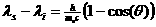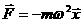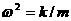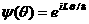Physics 401 Homework1---Due September 16

1.      Using the conservation of energy and momentum and assuming a two-body collision between a photon and an electron initially at rest, derive the Compton formula:where the subscripts i and s indicate the wavelength of the incident and scattered radiation respectively. (Hint: You may want to use relativity to first solve the problem in the center of mass frame and then boost. If you do not know relativeity well enough to do this uses brute force.).

2.      In class we discussed the Bohr atom. The derivation of the allowed energies was based on the electrostatic force, the quantization of angular momentum into integral units of Ñ and the assumption of circular orbits. Repeat this derivation supposing that the force holding a particle in its orbit is that of a three dimensional harmonic oscillator with a force given bywithwhere k is the spring constant and of the oscillator and m is the mass of the orbiting particle. Show the allowable radii for the circular orbits and the allowable energies. How do these allowable energies compare with those of Planck?

3.       Consider the following problem. A bead is constrained to move (without friction) along a wire. The wire is bent into a circular loop of radius R. Imposing the condition that the angular momentum is quantized into integral units of Ñ find the allowable energies.

4.       Let us try to gain some insights into the quantization of L in problem 3. Suppose, following de Broglie, that there is a wave function y which describes the system.  This wave function can be described in terms of q, the angular variable describing motion around the ring. By analogy with de Broglie’s description of linear motion one would expect the wave function for a state of good angular momentum to be. In deriving this we simply replace the ordinary position, x, by the angular position, q, and the ordinary momentum, p, by the angular momentum, L. In order that the wave function is well defined in the sense of being single-valued it must satisfy the condition y (q + 2p ) =y (q ) .  Show that this condition implies that L = n  Ñ with integral n.